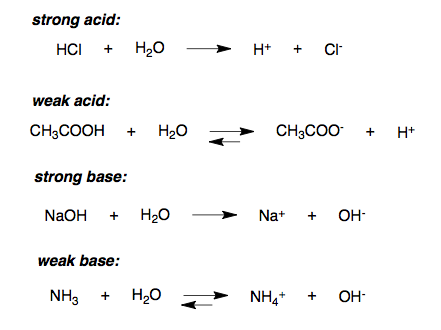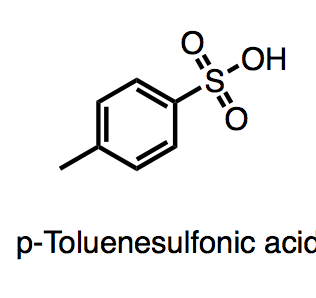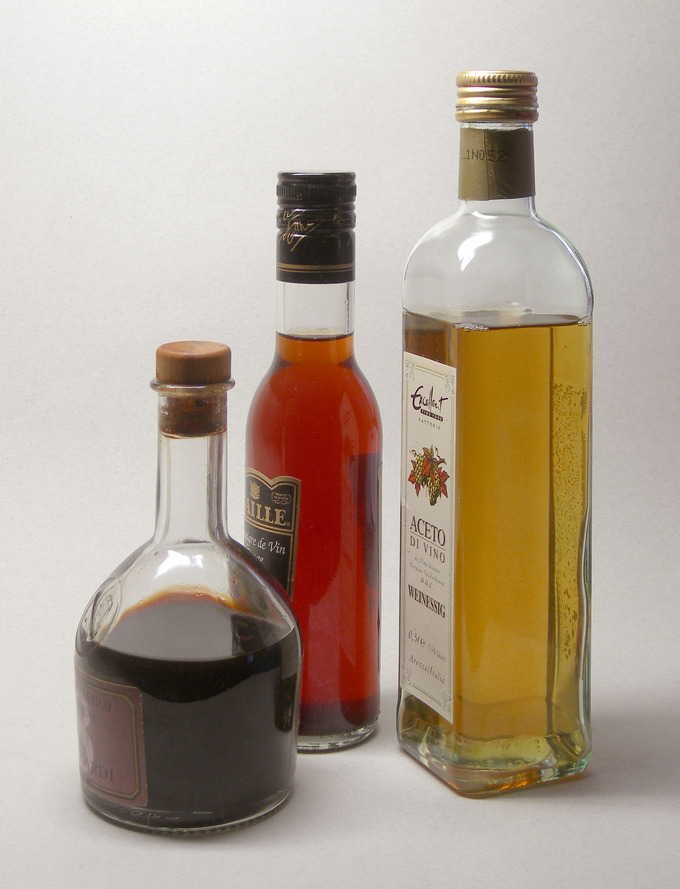Strong Acids

In water, strong acids completely dissociate into free protons and their conjugate base.

Learning Objectives

Calculate pH for solutions of strong acids.

Key Takeaways

Key Points

• Strong acids can catalyze chemical reactions.
• Strong acids are defined by their pKa. The acid must be stronger in aqueous solution than a hydronium ion, so its pKa must be lower than that of a hydronium ion. Therefore, strong acids have a pKa of <-174.
• Strong acids can be organic or inorganic.
• Strong acids must be handled carefully because they can cause severe chemical burns.
• Strong acids are essential for catalyzing some reactions, including the synthesis and hydrolysis of carbonyl compounds.

Key Terms

• carbonyl: a divalent functional group (-CO-), characteristic of aldehydes, ketones, carboxylic acids, amides, carboxylic acid anhydrides, carbonyl halides, esters, and others.
• ester: a compound usually formed by condensing an alcohol and an acid and eliminating of water. it contains the functional group carbon-oxygen double bond joined via carbon to another oxygen atom
• hydrolysis: a chemical process of decomposition; involves splitting a bond and adding the hydrogen cation and water’s hydroxide anion

Definition of Strong Acids

The strength of an acid refers to the ease with which the acid loses a proton. A strong acid ionizes completely in an aqueous solution by losing one proton, according to the following equation:

$\text{HA} (\text{aq}) \rightarrow \text{H}^+ (\text{aq}) + \text{A}^-(\text{aq})$

where HA is a protonated acid, H+ is the free acidic proton, and A is the conjugate base. Strong acids yield weak conjugate bases. For sulfuric acid, which is diprotic, the “strong acid” designation refers only to the dissociation of the first proton:

$\text{H}_2\text{SO}_4 (\text{aq}) \rightarrow \text{H}^+ (\text{aq}) + \text{HSO}_4 ^- (\text{aq})$

More precisely, the acid must be stronger in aqueous solution than a hydronium ion (H+), so strong acids have a pKa < -1.74. An example is hydrochloric acid (HCl), whose pKa is -6.3. This generally means that in aqueous solution at standard temperature and pressure, the concentration of hydronium ions is equal to the concentration of strong acid introduced to the solution.Ionization of acids and bases in water: A strong acid ionizes completely in an aqueous solution by losing one proton (H+).

Due to the complete dissociation of strong acids in aqueous solution, the concentration of hydronium ions in the water is equal to the total concentration (ionized and un-ionized) of the acid introduced to solution:

[H+] = [A] = [HA]total and pH = −log[H+].

Strong acids, like strong bases, can cause chemical burns when exposed to living tissue.

Examples of Strong Acids

Some common strong acids (acids with pKa < -1) include:

• Hydroiodic acid (HI): pKa = -9.3
• Hydrobromic acid (HBr): pKa = -8.7
• Perchloric acid (HClO4): pKa ≈ -8
• Hydrochloric acid (HCl): pKa = -6.3
• Sulfuric acid (H2SO4): pKa1 ≈ -3 (first dissociation only)
• p-Toluenesulfonic acid: pKa = -2.8
• Nitric acid (HNO3): pKa ≈ -1.4
• Chloric acid (HClO3): pKa ≈ 1.0p-Toluenesolfonic acid: p-Toluenesulfonic acid is an example of an organic soluble strong acid, with a pKa of -2.8.

Strong Acid Catalysis

Strong acids can accelerate the rate of certain reactions. For instance, strong acids can accelerate the synthesis and hydrolysis of carbonyl compounds. With carbonyl compounds such as esters, synthesis and hydrolysis go through a tetrahedral transition state, where the central carbon has an oxygen, an alcohol group, and the original alkyl group. Strong acids protonate the carbonyl, which makes the oxygen positively charged so that it can easily receive the double-bond electrons when the alcohol attacks the carbonyl carbon; this enables ester synthesis and hydrolysis.

Weak Acids

A weak acid only partially dissociates in solution.

Learning Objectives

Solve acid-base equilibrium problems for weak acids.

Key Takeaways

Key Points

• The dissociation of weak acids, which are the most popular type of acid, can be calculated mathematically and applied in experimental work.
• If the concentration and Ka of a weak acid are known, the pH of the entire solution can be calculated. The exact method of calculation varies according to what assumptions and simplifications can be made.
• Weak acids and weak bases are essential for preparing buffer solutions, which have important experimental uses.

Key Terms

• conjugate acid: the species created when a base accepts a proton
• conjugate base: the species created after donating a proton.
• weak acid: one that dissociates incompletely, donating only some of its hydrogen ions into solution

A weak acid is one that does not dissociate completely in solution; this means that a weak acid does not donate all of its hydrogen ions (H+) in a solution. Weak acids have very small values for Ka (and therefore higher values for pKa ) compared to strong acids, which have very large Ka values (and slightly negative pKa values).

The majority of acids are weak. On average, only about 1 percent of a weak acid solution dissociates in water in a 0.1 mol/L solution. Therefore, the concentration of H+ ions in a weak acid solution is always less than the concentration of the undissociated species, HA. Examples of weak acids include acetic acid (CH3COOH), which is found in vinegar, and oxalic acid (H2C2O4), which is found in some vegetables.Vinegars: All vinegars contain acetic acid, a common weak acid.

Dissociation

Weak acids ionize in a water solution only to a very moderate extent. The generalized dissociation reaction is given by:

$\text{HA}(\text{aq}) \rightleftharpoons \text{H}^+ (\text{aq}) + \text{A}^- (\text{aq})$

where HA is the undissociated species and A is the conjugate base of the acid. The strength of a weak acid is represented as either an equilibrium constant or a percent dissociation. The equilibrium concentrations of reactants and products are related by the acid dissociation constant expression, Ka:

$\text{K}_\text{a} = \frac{[\text{H}^+][\text{A}^-]}{[\text{HA}]}$

The greater the value of Ka, the more favored the H+ formation, which makes the solution more acidic; therefore, a high Ka value indicates a lower pH for a solution. The Ka of weak acids varies between 1.8×10−16 and 55.5. Acids with a Ka less than 1.8×10−16 are weaker acids than water.

If acids are polyprotic, each proton will have a unique Ka. For example, H2CO3 has two Ka values because it has two acidic protons. The first Ka refers to the first dissociation step:

$\text{H}_2\text{CO}_3 + \text{H}_2\text{O} \rightarrow \text{HCO}_3^{-} + \text{H}_3\text{O}^+$

This Ka value is 4.46×10−7 (pKa1 = 6.351). The second Ka is 4.69×10−11 (pKa2 = 10.329) and refers to the second dissociation step:

$\text{HCO}_3^- + \text{H}_2\text{O} \rightarrow \text{CO}_3^{2- } + \text{H}_3\text{O}^+$

Calculating the pH of a Weak Acid Solution

The Ka of acetic acid is $1.8\times 10^{-5}$. What is the pH of a solution of 1 M acetic acid?

In this case, you can find the pH by solving for concentration of H+ (x) using the acid’s concentration (F) and Ka. Assume that the concentration of H+ in this simple case is equal to the concentration of A, since the two dissociate in a 1:1 mole ratio:

$\text{K}_\text{a} = \frac{[\text{H}^+][\text{C}_2\text{H}_3\text{O}_2^-]}{[\text{HA}]} = \frac{\text{x}^2}{(\text{F}-\text{x})}$

This quadratic equation can be manipulated and solved. A common assumption is that x is small; we can justify assuming this for calculations involving weak acids and bases, because we know that these compounds only dissociate to a very small extent. Therefore, our above equation simplifies to:

$\text{K}_\text{a}=1.8\times 10^{-5}=\frac{\text{x}^2}{\text{F}-\text{x}}\approx \frac{\text{x}^2}{\text{F}}=\frac{\text{x}^2}{1\text{ M}}$

$1.8\times 10^{-5}=\text{x}^2$

$\text{x}=3.9 \times 10^{-3}\text{ M}$

$\text{pH}=-\text{log}[\text{H}^+]=-\text{log}(3.9\times 10^{-3})=2.4$

Although it is only a weak acid, a concentrated enough solution of acetic acid can still be quite acidic.

Calculating Percent Dissociation

Percent dissociation represents an acid’s strength and can be calculated using the Ka value and the solution’s pH.

Learning Objectives

Calculate percent dissociation for weak acids from their Ka values and a given concentration.

Key Takeaways

Key Points

• Percent dissociation is symbolized as α ( alpha ) and represents the ratio of the concentration of dissociated hydrogen ion [H+] to the concentration of the undissociated species [HA].
• Unlike Ka, percent dissociation varies with the concentration of HA; dilute acids dissociate more than concentrated ones.
• Percent dissociation is related to the concentration of both the conjugate base and the acid ‘s initial concentration; it can be calculated if the pH of the solution and the pKa of the acid are known.

Key Terms

• dissociation: the process by which compounds split into smaller constituent molecules, usually reversibly.
• percent ionization: the fraction of an acid that undergoes dissociation

We have already discussed quantifying the strength of a weak acid by relating it to its acid equilibrium constant Ka; now we will do so in terms of the acid’s percent dissociation. Percent dissociation is symbolized by the Greek letter alpha, α, and it can range from 0%< α < 100%. Strong acids have a value of α that is equal to or nearly 100%; for weak acids, however, α can vary, depending on the acid’s strength.

Example

Calculate the percent dissociation of a weak acid in a $0.060\;\text{M}$ solution of HA ($\text{K}_\text{a}=1.5\times 10^{-5}$).

To determine percent dissociation, we first need to solve for the concentration of H+. We set up our equation as follows:

$\text{K}_\text{a}=\frac{[\text{H}^+][\text{A}^-]}{[\text{HA}]}$

$1.5\times 10^{-5}=\frac{\text{x}^2}{0.060-\text{x}}$

However, because the acid dissociates only to a very slight extent, we can assume x is small. The above equation simplifies to the following:

$1.5\times 10^{-5}\approx \frac{\text{x}^2}{0.060}$

$\text{x}=[\text{H}^+]=9.4\times 10^{-4}$

To find the percent dissociation, we divide the hydrogen ion’s concentration of by the concentration of the undissociated species, HA, and multiply by 100%:

$\alpha = \frac{[\text{H}^+]}{[\text{HA}]}\times 100\%=\frac{9.4\times 10^{-4}}{0.060}\times 100\%=1.6\%$

As we would expect for a weak acid, the percent dissociation is quite small. However, for some weak acids, the percent dissociation can be higher—upwards of 10% or more. For example, with a problem involving the percent dissociation of a 0.100 M chloroacetic acid, we cannot assume x is small, and therefore use an ICE table to solve the problem.

Ka from Percent Dissociation: In this video, the percent dissociation of the acid is significant (11%), and se we cannot assume x is small. An ICE chart is used to calculate the relevant concentrations.